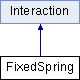ParM  parm A molecular dynamics library
FixedSpring Class Reference

#include <interaction.hpp>

Inheritance diagram for FixedSpring:## Public Member Functions

FixedSpring (vector< FixedSpringAtom > atoms=vector< FixedSpringAtom >())

void add (FixedSpringAtom a)

void add (AtomID a, Vec loc, flt k, bool usex=true, bool usey=true, bool usez=true)

uint size () const

flt energy (Box &box)
Potential energy due to this Interaction. More...

void set_forces (Box &box)

flt pressure (Box &box)
Partial pressure due to this Interaction. More...Public Member Functions inherited from Interaction
virtual flt set_forces_get_pressure (Box &box)
Set forces (Atom.f) and return $$P = \sum_{\left<i,j \right>} \vec r_{ij} \cdot \vec F_{ij}$$ at the same time (see pressure()). More...

virtual Matrix stress (Box &box)
The force-moment tensor for the current simulation: More...

virtual ~Interaction ()

## Protected Attributes

vector< FixedSpringAtomatoms

## Constructor & Destructor Documentation

 FixedSpring::FixedSpring ( vector< FixedSpringAtom > atoms = vector() )
inline

## Member Function Documentation

 void FixedSpring::add ( FixedSpringAtom a )
inline
 void FixedSpring::add ( AtomID a, Vec loc, flt k, bool usex = true, bool usey = true, bool usez = true )
inline
 flt FixedSpring::energy ( Box & box )
inlinevirtual

Potential energy due to this Interaction.

Implements Interaction.

 flt FixedSpring::pressure ( Box & box )
inlinevirtual

Partial pressure due to this Interaction.

$$P = \sum_{\left<i,j \right>} \vec r_{ij} \cdot \vec F_{ij}$$, or equivalently $$P = \sum_i \vec r_i \cdot \vec F_i$$

Note that the full pressure involves all interactions and temperature, and needs to be normalized by $$\frac{1}{dV}$$ where $$d$$ is the number of dimensions and $$V$$ is the volume.

Implements Interaction.

 void FixedSpring::set_forces ( Box & box )
inlinevirtual

Implements Interaction.

 uint FixedSpring::size ( ) const
inline

## Member Data Documentation

 vector FixedSpring::atoms
protected

The documentation for this class was generated from the following file: